# Round Time to Nearest 15 Minutes (or Other Multiple) – Excel & Google Sheets

This tutorial will demonstrate how to round time in Excel and Google Sheets.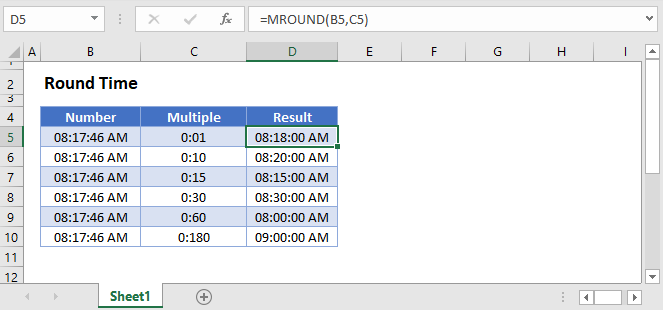## Round Time

To round time in Excel, you should use the MROUND Function, entering your rounding multiple as a unit of time (surrounded by quotations).

``=MROUND(B3,"0:15")``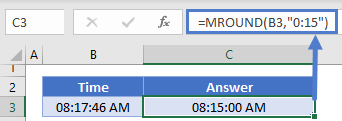This example rounded time to the nearest 15 minutes. Instead you could round to the nearest 60 minutes instead.

``=MROUND(B3,"0:60")``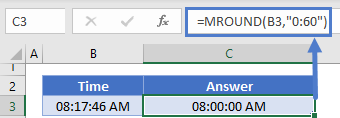Another way to enter time is by using decimal values. In Excel, time is stored as decimal values.

For example, 1/24 represents one hour, by entering 1/24 into MROUND, you will round to the nearest hour.

``=MROUND(B3,1/24)``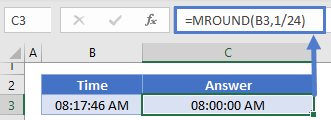## Round Time Up

To round time up, use the exact same technique except with the CEILING Function.

``=CEILING(B3,"0:15")``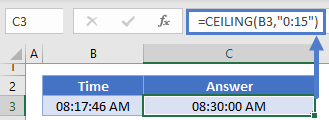## Round Time Down

To round time down, use the exact same technique except with the FLOOR Function.

``=FLOOR(B3,"0:15")``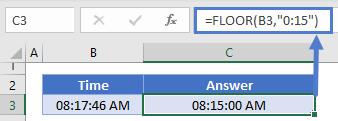## Round Time in Google Sheets

All of the above examples work exactly the same in Google Sheets as in Excel.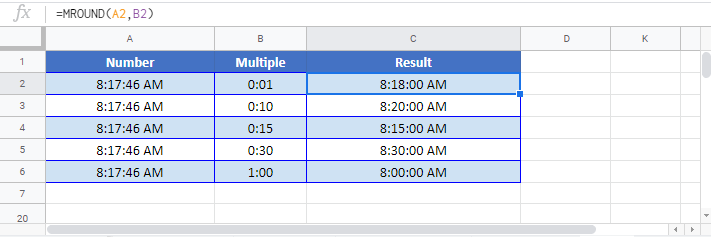### Excel Practice Worksheet

Practice Excel functions and formulas with our 100% free practice worksheets!